I’ve written several times about the “squircle,” a sort of compromise between a square and a circle. It looks something like a square with rounded corners, but it’s not. Instead of having flat sizes (zero curvature) and circular corners (constant positive curvature), the curvature varies continuously.

A natural question is just what kind of circle approximates the corners. This post answers that question, finding the radius of curvature of the osculating circle.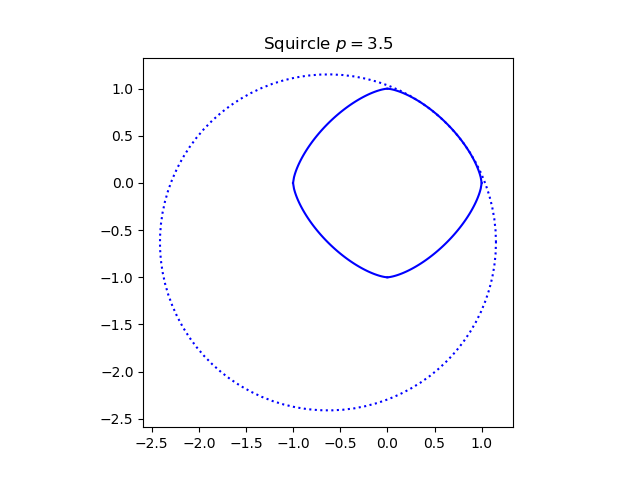The squircle has a parameter p which determines how close the curve is to a circle or a square.The case p = 2 corresponds to a circle, and in the limit as p goes to infinity you get a square.

We’ll work in the first quadrant so we can ignore absolute values. The curvature at each point is complicated  but simplifies in the corner toand the radius of curvature is the reciprocal of this. So for moderately large p, the radius of curvature is approximately √2/(p-1).

In the image at the top of the post, p = 3.5. Here’s an image with a larger value of p, p = 10.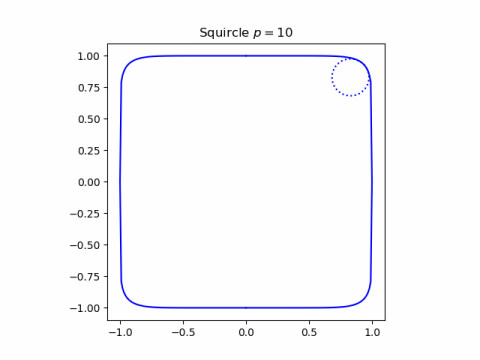And here’s one with a smaller value, p = 2.5.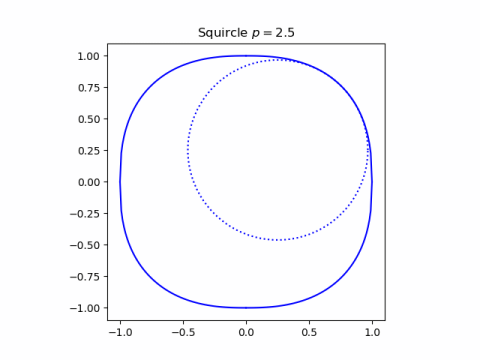When p = 2 we get a circle. When p is between 1 and 2 we get more of a diamond than a square. Notice in the image below with p = 1.5 the osculating circle is larger than the squircle, and the “corner” is nearly the whole side.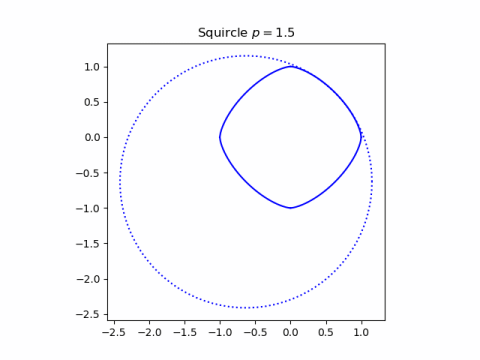Finally, for p between 0 and 1 the sides of the diamond cave in giving a concave shape. Now the osculating circle is on the outside.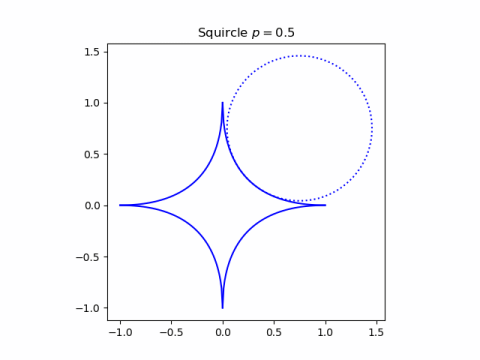## Related posts

 The general expression is